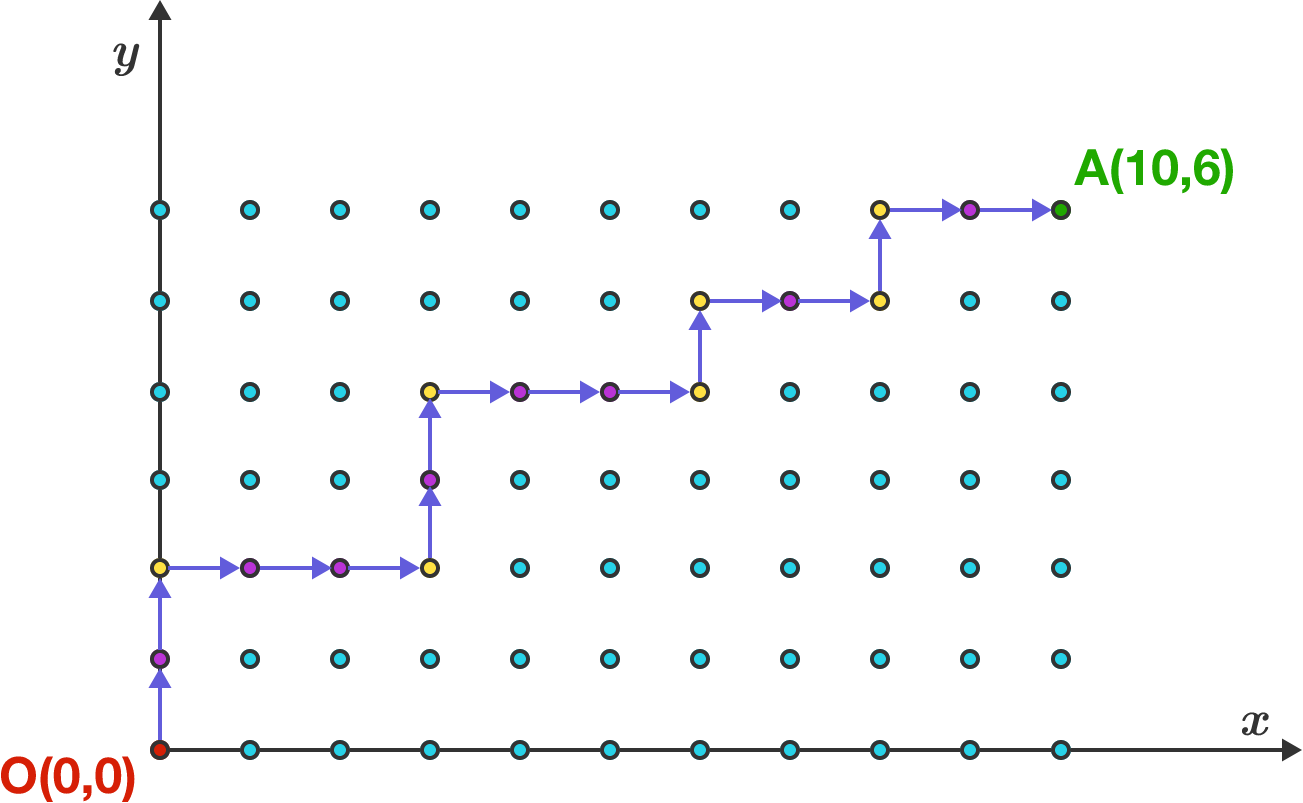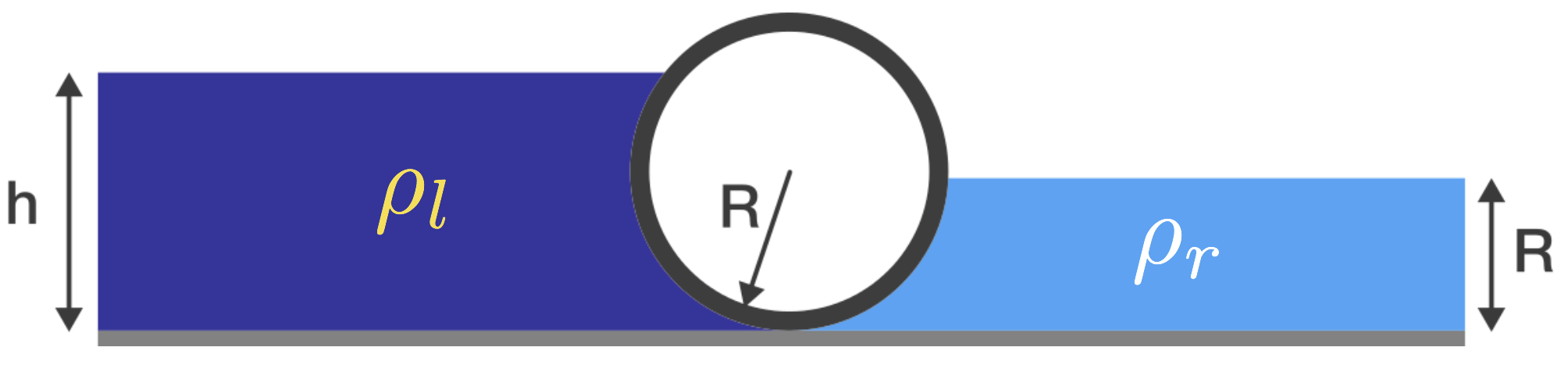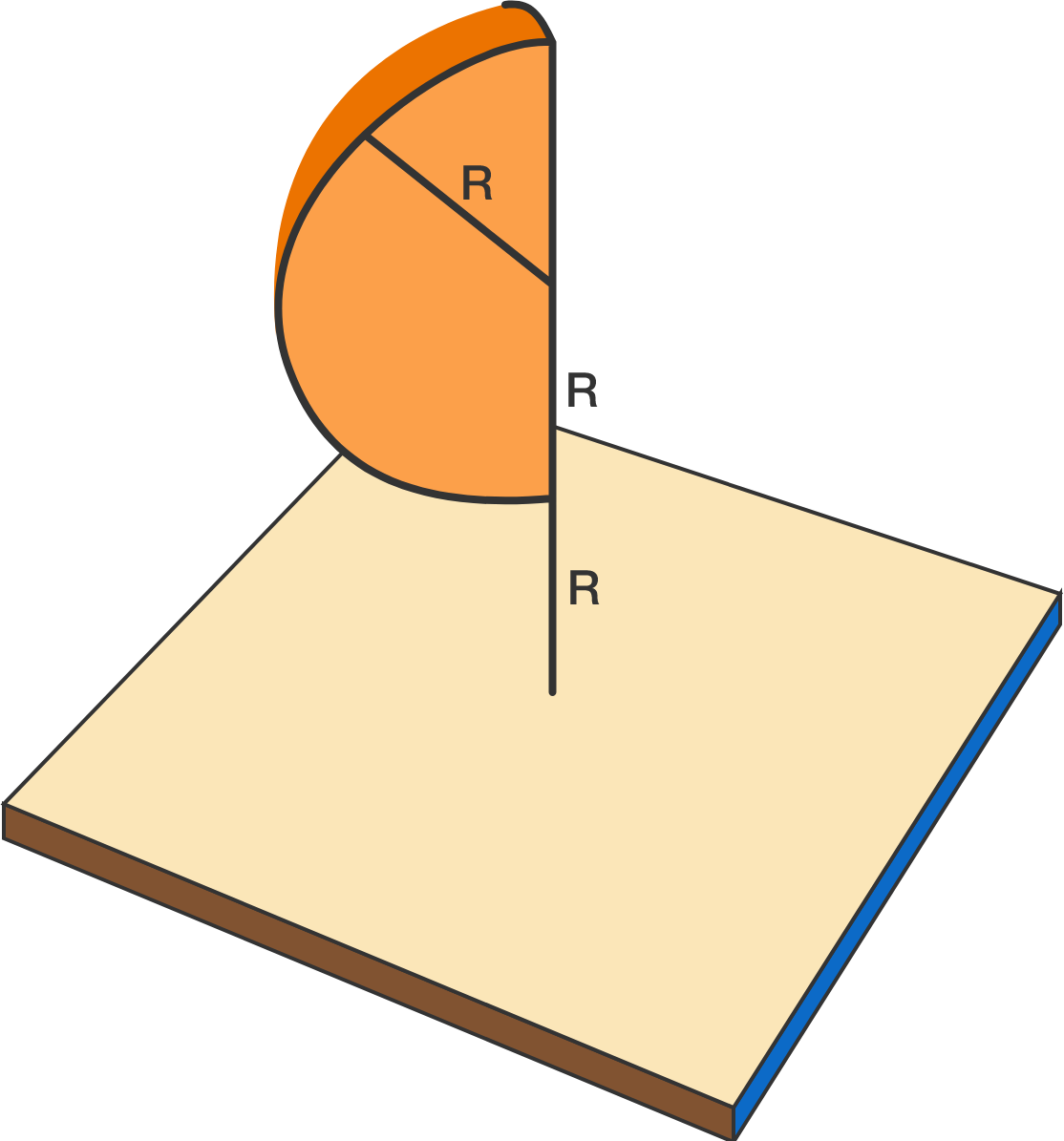# Problems of the Week

Contribute a problem

True or False?

Given any six irrational numbers, there always exist three such that the sum of any two of them is still irrational.Richard P Feynman starts his journey from $O=(0,0)$ towards $A=(10,6).$ He can only go in the $+x$ and $+y$ directions along the lattice, and he must turn exactly 7 times. An example path is shown above, with the yellow dots indicating turns.

How many ways can Feynman travel from $O$ to $A$ in this way?

Bonus: Consider the general case, traveling from $(0,0)$ to $(p,q)$ with exactly $r$ turns.A heavy cylinder of radius $R$ lies in equilibrium on a smooth surface, separating two liquids of densities $\rho_l$ on the left and $\rho_r$ on the right, with $\rho_r > \rho_l.$

If the height of the liquid on the right is $R,$ what is the height $h$ of the liquid on the left in terms of $R?$

Neglect the surface tensions of the liquids.

Find the smallest positive real $x$ such that $\big\lfloor x^2 \big\rfloor-x\lfloor x \rfloor=6.$ If your answer is in the form $\frac{a}{b}$, where $a$ and $b$ are coprime positive integers, submit your answer as $a+b.$


Notation: $\lfloor \cdot \rfloor$ denotes the floor function.A uniform solid in the shape of a quarter segment of a sphere of radius $R$ is released from rest with its diameter vertical, and the center a height of $2R$ above a smooth horizontal floor. The solid strikes the floor in a perfectly elastic collision. The angular velocity of the segment, immediately after its collision with the floor, is of the form $\omega \; = \; \alpha\sqrt{\frac{g}{R}}$ for some $\alpha > 0$, where $g$ is the constant of gravitational acceleration.

What is the value of $\alpha$ to $2$ decimal places?

Inspiration

×